# Mapping cylinder

This article describes a construct that involves some variant of taking a product of a topological space with the unit interval and then making some identifications, typically at the endpoints, based on some specific maps.
View more such constructs

## Definition

Let$f:X \to Y$ be a function. Then the mapping cylinder of$f$ is defined as the quotient of the disjoint union of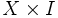$X \times I$ with$Y$, modulo the equivalence relation: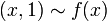$\! (x,1) \sim f(x)$

Here,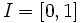$I = [0,1]$ is the unit interval.

## Facts

The significance of the mapping cylinder is that it is homotopy-equivalent to$Y$, and moreover the inclusion of$X$ (say via$x \mapsto (x,0)$) in the mapping cylinder is equivalent to the map$f$.

Thus, starting from an arbitrary continuous map, we have got a homotopy-equivalent map which is an inclusion.

Further, the inclusion of$X$ in the mapping cylinder is a cofibration, which makes it even nicer.

## Relation with other constructions

### More general constructions

Name of construction Description of construction How the mapping cylinder is a special case
double mapping cylinder spaces$X,Y,Z$, with continuous maps from$X$ to$Y$ and$X$ to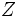$Z$, we take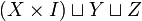$(X \times I) \sqcup Y \sqcup Z$ and collapse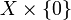$X \times \{ 0 \}$ and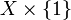$X \times \{ 1 \}$ onto$Z$ and$Y$ via the continuous maps Case where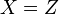$X = Z$ and the map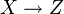$X \to Z$ is the identity map.

### More specific constructions

Name of construction How it arises as a special case
cone space Set$Y$ as a one-point space and$f:X \to Y$ as the map sending everything to one point.